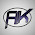# State the Universal law of Gravitation :

Hello Readers here you will read State the Universal law of Gravitation. The law is applicable to all the objects in Universe , such a law is said to be Universal.

State the Universal law of Gravitation :-

Every object in the Universe attracts every other object with a force which is proportional to the product of their masses and inversely proportional to the square of the distance between them. The force is along the line joining the centres of two objects.

Suppose the M mass of an object

and m is the mass of the second object

And they are located at a distance d from each other.

Let the force of attraction between two objects be F. According to the universal law of gravitation, the force between two objects is directly proportional to the product of their masses. That is,

F  ∝ M × m    - - - - - - - (1)

And the force between two objects is inversely proportional to the square of the distance between them, that is,

1/ d2      - - - - - - - (2)

Combining 1 and 2

F   ∝  M × m / d2     - - - - - - - (3)

or F = G M × m / d2     - - - - - - - (4)

First, Where G is the constant of proportionality  Second it is called the universal gravitation constant.

By multiplying crosswise Equation 4 gives

F × d2 = G M × m

or G = F d2 / M × m    - - - - - - - (5)

The law is applicable to all the bodies.

State the Universal law of Gravitation , What is the Universal law of Gravitation

1.